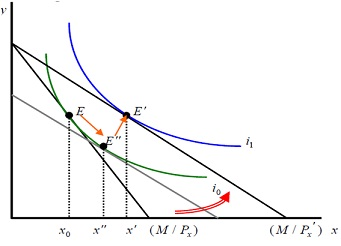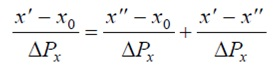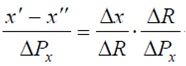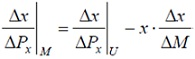#### Change in Price, Slutzky Equation and Labor Supply application

Change in Price: Revisited

* Price Effect (E → E′)

(E→E′′): Substitution Effect due to price change (E′′→ E′):Income Effect

In this case, good x is a normal good.

cf) Derive substitution as well as income effect if good x is neither a normal nor an inferior good.
cf) Derive substitution and income effect if good x is an inferior good. Maybe you can think of two different cases.Slutzky Equation (Slutzky Decomposition)

x′ − x0 = (x′′ − x0 ) + (x′ − x′′) .............(1)

Dividing both sides by ΔPx , we get the following equation,...........(2)

l.h.s. can be explained (Δx/ΔPx)|M And the first term on r.h.s. is equivalent to (Δx/ΔPx)|U

As well as the second term on r.h.s. is now revised as follows:...........(3)

where R means real income.

ΔR/ΔPx means the change in real income with response to change in price.

So, ΔR/ΔPx = -x (Shepard’s lemma)..........(4)
And Δx/ΔR=Δx/ΔM (why? assume about the definition of income effect!)

Plugging (4) and (5) in (3) and then in (2), we can get: (Slutzky Equation)Labor Supply and Leisure (Application)

H + L = 24, where H is hours worked and L is amount of leisure activities.

And hourly wage is given at w0 as well as non-labor income is given at V0.

The utility function is now U = U(L, M)............(1)

The budget equation is M = w0H + V0 = w0 (24 − L) + V0 = 24w0 − w0L + V0 .............(2)

Rewriting (2), we can get M + w0L = 24w0 + V0 (full-income constraint) .............(3)

If we assume the price level of goods (composite good) is PC = \$1, then M on l.h.s would equal total number of goods that this consumer buys.

Can you interpret terms in equation (3)?

1) Now can you depict the budget line?
2) Find the utility-maximizing point.
3) Suppose wage increases. Overlap fresh optimal points on the original budget line.
4) Connect those optimization points to obtain PCC And labour supply curve.
5) Why do you suppose is it a backward bending supply curve?

Latest technology based Microeconomics Online Tutoring Assistance

Tutors, at the www.tutorsglobe.com, take pledge to provide full satisfaction and assurance in Microeconomics help via online tutoring. Students are getting 100% satisfaction by online tutors across the globe. Here you can get homework help for Microeconomics, project ideas and tutorials. We provide email based Microeconomics help. You can join us to ask queries 24x7 with live, experienced and qualified online tutors specialized in Microeconomics. Through Online Tutoring, you would be able to complete your homework or assignments at your home. Tutors at the TutorsGlobe are committed to provide the best quality online tutoring assistance for Microeconomics Homework help and assignment help services. They use their experience, as they have solved thousands of the Microeconomics assignments, which may help you to solve your complex issues of Microeconomics. TutorsGlobe assure for the best quality compliance to your homework. Compromise with quality is not in our dictionary. If we feel that we are not able to provide the homework help as per the deadline or given instruction by the student, we refund the money of the student without any delay.